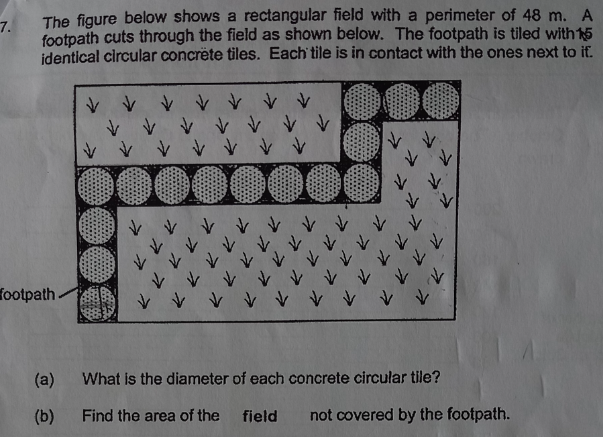# QuestionThank you!

Source: ?

This question is not well designed.  The words and the picture is confusing.   Are we supposed to think the footpath is made of circles or has a rectangular skirting around the circles?

So should I assume it with the skirting since there is no π value given?  Typically a question with circle calculation would provide the π value.   Like the previous question no π value was given because there was no need to use the circle formula at all.

d = diameter of circle

2(10+6)d = 48

d = 48/32 = 1.5m

(b)  Area of rectangular field = (10 x 1.5) x (6 x 1.5) = 135

Area of the footpath = (4.5  + 1.5  + 12  + 4.5 ) x 1.5 = 33.75

Hence area of field not covered by the footpath = 135-33.75 = 101.25cm2

PS:  Please let me know if the answer is 101.25 or 108.5(to 1 dec place).

2 Replies 1 Like ✔Accepted Answer

Area of the footpath = (4.5  + 1.5  + 12  + 4.5 ) x 1.5 = 33.75

For this step, why must x1.5?